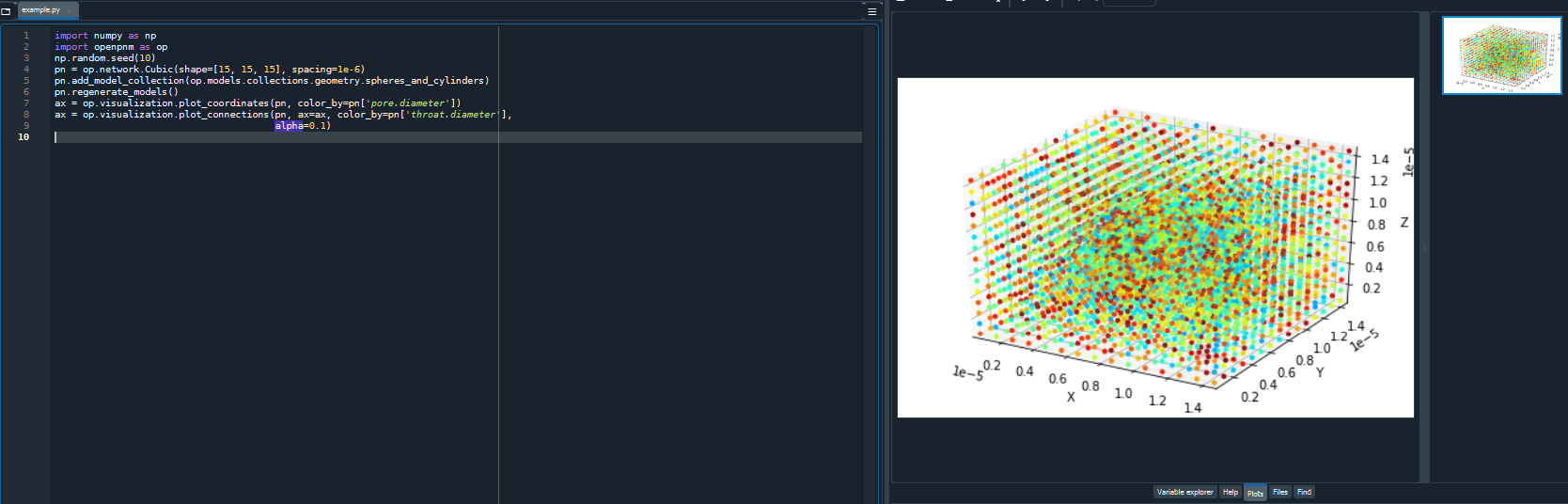# Visualization options#

Different options are available to visualize a pore network. In this notebook we cover plotting in Spyder,interactive plots in Jupyter, and using Paraview software to visualize the network and simulation results.

%config InlineBackend.figure_formats = ['svg']
%matplotlib inline
import os
current_directory=os.getcwd()


## Spyder#

Spyder is an open-source IDE for Python. The Plots pane in Spyder shows generated images and plots in Console. For more details see Spyder Plots. As an example, let’s run the following codes in Spyder’s Editor and visualize the results:

import numpy as np
import openpnm as op
import matplotlib.pyplot as plt
np.random.seed(10)


We create a cubic network and visualize the network pores and throats using plot_coordinates and plot_connections. These functions are based on matplotlib functions and costumized for pore network visualization. Here the pores and throats are colored by the pores and throats diameter. The throats are shown as connections in a wireframe. We used alpha to create a more transparent color for throats. :

pn = op.network.Cubic(shape=[15, 15, 15], spacing=1e-6)
pn.regenerate_models()
ax = op.visualization.plot_coordinates(pn, color_by=pn['pore.diameter'])
ax = op.visualization.plot_connections(pn, ax=ax, color_by=pn['throat.diameter'], alpha=0.1)The results from running in Spyder:Other types of plots and examples such as histograms of data, plot coordinates and connections for extracted network, distribution of quantities in phase, etc can be generated and visualized in Spyder.

## Interactive plots in Jupyter notebook#

The plots in Spyder and Jupyter notebook shown in previous examples are static.To create an interactive plot in Jupyter notebook we use Plotly Python, which is an interactive open-source library for plotting in Python. Plotly has different methods to plot data such as histogram, scatter plot, etc. When using Jupyter notebooks for pore network visualization, OpenPNM’s costumized function plot_notebook (based on Plotly) can be used. Following shows an example. The resulting plot is interactive to (rotate, zoom, etc) and by hovering over pores their indice and coordinates will be shown in a box.

ax = op.visualization.plot_notebook(pn, node_color=pn['pore.diameter'], node_scale=10)
ax.show()